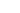### Tag: rng## A fast random number generator in CIf you are using RNGs (Random Number Generators) for cryptography then you need one that has been validated for sufficient randomness. For example the libsodium library.

But if you want a fast one for general purpose use, then xoshiro256++ is a fast one.  It’s actually that short that I can list it here. Just call next() and mod it (%) with the highest value+1. E.G. for a dice roll,

``````int diceroll= (next() %6) +1;
``````

This algorithm is very fast, typically on the order of nano-seconds 10-9 seconds.

``````#include <stdint.h>
uint64_t rngstate;

static inline uint64_t rotl(const uint64_t x, int k) {
return (x << k) | (x >> (64 - k));
}

// Returns a Uint64 random number
uint64_t next(void) {
const uint64_t result = rotl(rngstate + rngstate, 23) + rngstate;
const uint64_t t = rngstate << 17;
rngstate ^= rngstate;
rngstate ^= rngstate;
rngstate ^= rngstate;
rngstate ^= rngstate;
rngstate ^= t;
rngstate = rotl(rngstate, 45);
return result;
}``````## Fast Random Number Generators in CToday I found out about Romu (Rotate and Multiply) random number generators. Typically I’ve been using srand() in my asteroids game, so this is well worth a look.  Their code page lists functions, so they should be easy to plugin.